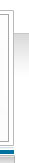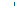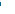ClassicalRealAnalysis.info
Serving the mathematics community.Homedriptopten-10
 #10.   Well...it's just so "uniformly" bad!   Indeed that's the problem. It is a uniform definition that many insist on teaching in an era when a pointwise definition is universally recognized as providing the correct theory of integration. A function f(x) is integrable on [a,b] if for everyε>0 there is a function δ:[a,b] -> (0,1) so that when a partition is finer than δ then the Riemann sum is ... A function f(x) is Riemann integrable on [a,b] if for every ε>0 there is a constant function δ:[a,b] -> (0,1) so that when a partition is finer than δ then the Riemann sum is ... Why teach the uniform version? Well if you insist on it. Then perhaps you should also teach the uniform derivative too: A function F on [a,b] has a uniform derivative F'(x) = lim [F(x+h)-F(x)/h if this limit exists uniformly for x in [a,b] as h->0. Here is a completely trivial theorem. If F has a uniform derivative then F' is Riemann integrable. That's much easier than the usual version in calculus where you assume Riemann integrability and use the mean-value theorem. Here's two more trivial theorems, with almost exactly the same proofs: If f is continuous at each point of [a,b] then f is integrable. If f is uniformly continuous on [a,b] then f is Riemann integrable. But don't try proving that continuous functions are Riemann integrable. That's way harder. You have to first prove that continuous functions are uniformly continuous. Indeed here is the problem: to use the Riemann integral you are always obliged to check for a uniform condition. This imposes a burden in every proof, either a burden within the proof or a burdensome extra hypothesis. Teaching the Riemann integral quickly opens up this little puzzle of pointwise vs uniform conditions. Once this door is open you may as well come clean and own up to the existence of a nonuniform version of the integral that is universally used. Push that door shut to keep out any more of the theory than you are willing to teach, but don't suppress the whole story. You can tell them that the best way to teach this integral is not with this simple definition but by developing Lebesgue's theory of measure, and that only graduate students are considered mature enough to handle such sophisticated ideas. (It's a load of rubbish, but some people apparently believe it.) Posted by BST at 4:28 PM January 15, 2008     Top ten reasons for dumping the Riemann integral:  #10, #9, #8, #7, #6, #5, #4, #3, #2, #1pro quo hic escorol. Olypian quarrels et gorilla congolium sic ad nauseum. Souvlaki ignitus carborundum e pluribus unum. Defacto lingo est igpay atinlay. Marquee selectus non provisio incongruous feline nolo contendre. Gratuitous octopus niacin, sodium glutimate.Olypian quarrels03/05/07 Pro quo hic escorol. Olypian quarrels et gorilla congolium sic ad nauseum.03/05/07 Pro quo hic escorol. Olypian quarrels et gorilla congolium sic ad nauseum.03/05/07 Pro quo hic escorol. Olypian quarrels et gorilla congolium sic ad nauseum.